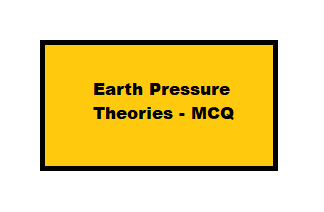# Earth Pressure Theories – MCQ || Foundation Engineering ||

3rd July 2020

## Earth Pressure Theories – MCQ

1. Depth of tensile zone near the surface of a cohesive fill is –

a)$(c/\gamma )/ N\phi$

b)$(\gamma/c )/ N\phi$

c)$(2c/\gamma )/ N\phi$

d)$(2c/\gamma )/ (1/ N\phi)$

2. A verticle cut in clay soil with unit weight of 19 KN/m3 failed when the depth of cut was 4.8 m. What is the cohesive strength of clay?

a) 22 KN/m2

b) 20 KN/m2

c) 21 KN/m2

d) 23 KN/m2

[Note: Formula,$H_{c} = 4c/\gamma$ Where, H = 4.8m, c = cohesive strength of clay, and$\gamma$ = 19 KN/m3 ]

3. Which Assumption is true for the Rankine Earth pressure Theory –

a) The soil mass is dry and cohesionless

b) The soil mass is semi-infinite and homogeneous

c) The back of the wall is verticle and smooth

d) All of These ✅

4. The lateral earth pressure coefficient Kp and Ka refers to

a) Total stree

b) Natural Stress

c) Effective Stress ✅

d) All of these

e) None of these

5. A verticle cut in soft clay be excavated without any lateral support up to a depth of

a)$2c/\gamma$

b)$2c\sqrt{N_{\phi }}/\gamma$

c)$4c\sqrt{N_{\phi }}/\gamma$

d)$4c/\gamma$

6. Which condition fulfil the Coefficient of earth pressure at rest –

a) Greater than active earth pressure

b) Less than the Active earth pressure

c) Greater than the passive earth pressure

d) Less than the passive earth pressure

e) (a) but (d) ✅

f) (b) but (c)

7. Accoring to Rankine’s theory the value of ‘c’ for cohesionless soil is

a) 1

b) 0 ✅

c) 1/2

d) Non of These

8. Which Assumption is true for the Coulom’s Wedge Theory

a) The back fill is dry, cohesionless, homogenous

b) The back fill is elastically undeformable

c) All of These ✅

d) None of These